Next: Calculation of specific heats Up: Classical thermodynamics Previous: The equation of state

## Heat capacity or specific heat

Suppose that a body absorbs an amount of heat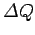and its temperature consequently rises by. The usual definition of the heat capacity, or specific heat, of the body is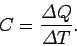(285)

If the body consists ofmoles of some substance then the molar specific heat (i.e., the specific heat of one mole of this substance ) is defined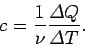(286)

In writing the above expressions, we have tacitly assumed that the specific heat of a body is independent of its temperature. In general, this is not true. We can overcome this problem by only allowing the body in question to absorb a very small amount of heat, so that its temperature only rises slightly, and its specific heat remains approximately constant. In the limit as the amount of absorbed heat becomes infinitesimal, we obtain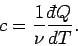(287)

In classical thermodynamics, it is usual to define two specific heats. Firstly, the molar specific heat at constant volume, denoted(288)

and, secondly, the molar specific heat at constant pressure, denoted(289)

Consider the molar specific heat at constant volume of an ideal gas. Since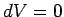, no work is done by the gas, and the first law of thermodynamics reduces to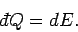(290)

It follows from Eq. (288) that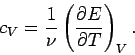(291)

Now, for an ideal gas the internal energy is volume independent. Thus, the above expression implies that the specific heat at constant volume is also volume independent. Sinceis a function only of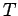, we can write(292)

The previous two expressions can be combined to give(293)

for an ideal gas.

Let us now consider the molar specific heat at constant pressure of an ideal gas. In general, if the pressure is kept constant then the volume changes, and so the gas does work on its environment. According to the first law of thermodynamics,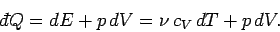(294)

The equation of state of an ideal gas tells us that if the volume changes by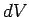, the temperature changes by, and the pressure remains constant, then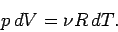(295)

The previous two equations can be combined to give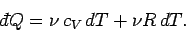(296)

Now, by definition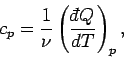(297)

so we obtain(298)

for an ideal gas. This is a very famous result. Note that at constant volume all of the heat absorbed by the gas goes into increasing its internal energy, and, hence, its temperature, whereas at constant pressure some of the absorbed heat is used to do work on the environment as the volume increases. This means that, in the latter case, less heat is available to increase the temperature of the gas. Thus, we expect the specific heat at constant pressure to exceed that at constant volume, as indicated by the above formula.

The ratio of the two specific heatsis conventionally denoted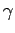. We have(299)

for an ideal gas. In fact,is very easy to measure because the speed of sound in an ideal gas is written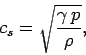(300)

where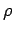is the density. Table 2 lists some experimental measurements ofandfor common gases. The extent of the agreement betweencalculated from Eq. (299) and the experimentalis quite remarkable.

Table 2: Specific heats of common gases in joules/mole/deg. (at 15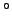C and 1 atm.) From Reif.
 Gas Symbol(experiment) (experiment) (theory) Helium He 12.5 1.666 1.666 Argon Ar 12.5 1.666 1.666 Nitrogen20.6 1.405 1.407 Oxygen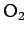21.1 1.396 1.397 Carbon Dioxide28.2 1.302 1.298 Ethane39.3 1.220 1.214Next: Calculation of specific heats Up: Classical thermodynamics Previous: The equation of state
Richard Fitzpatrick 2006-02-02